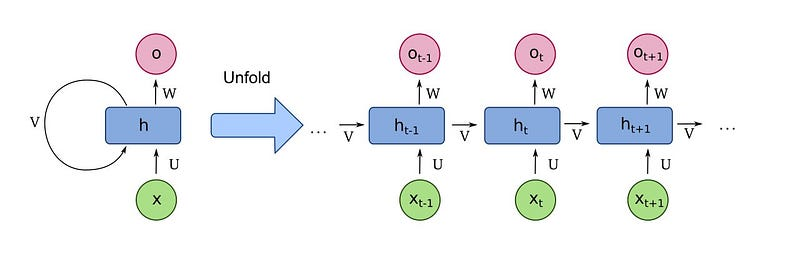Photo by Jez Timms on Unsplash

In this article, we’ll be learning about using machine learning on sequential data. Recurrent Neural Networks (RNNs) and Long Short Term Memory (LSTM) are two types of networks that could be used for this purpose. Lastly, we’ll implement one TensorFlow model from scratch using the IMDB dataset.

Its applications are very wide including chatbots, translators, text generators, sentiment analysis, speech recognition and so on…

### Introduction

First, let’s understand sequential data in detail so that we are on the same page. Sequential data could be any data that is dependent on the previous version of it. For example, text data in communication would require an understanding of a topic in a sequential manner. Another good example is sound data, as we need to remember what someone said earlier to understand the context of the current discussion. So such types of models are highly dependent on the sequence of the data and little tweaks in sequence could show high changes to accuracy.

### Prerequisites

• Basic understanding of neural networks
• Basic understanding of Natural Language Processing (NLP)
• Tensorflow 2.0
• Python3

### RNNs

This type of network has a block of function which receives two inputs, activation and input data and returns an output. Again the network is passing that output to the same block with the next input data recurrently until all the input data are used. Hence, its name is Recurrent neural network. It could be understood better from the following figure:Source

Diving into mathematical representations is beyond the scope of this tutorial but if you want to learn that you can refer to this video. But this model has one drawback with learning long sequences.

Suppose there is a sentence like this, “Neil was an astronaut. He was also the first person to land on the moon.”. Here, we can clearly understand that ‘He’ in the second sentence represents Neil but that information is not conveyed in RNNs. To tackle this situation we’ll understand GRU.

### GRU

As we’d seen above, RNN is not able to memorize the context of conversation which is not suitable for real-time usage. So as a solution to this Gated Recurrent Unit (GRU) was represented. It has a memory cell unit to remember the context of previous sequences. To understand GRU in detail refer to this video.

### LSTM

LSTM stands for Long Short Term Memory. It is even an advanced version of GRU. Even though it was invented way before GRU, the complexity of it is higher than GRU. It has multiple gates to handle different parameters. But this increases the calculation overhead on the model and is slow to train compared to GRU which could be considered as a trade-off for high accuracy. To understand the mathematics of LSTM refer to this video.

### Types of models

These models are categorized into three categories based on the inputs and outputs. They are classified as below:

1. Many-Many models: These models have multiple inputs and multiple outputs. Translators are one of the examples of such models.
2. One-Many models: These models have a single input and multiple outputs. Some text generators are such to generate the sequence of text from a single word.
3. Many-one models: These models have multiple inputs and a single output. Sentiment analysis is an example of such a model that takes a sequence of review text as input and outputs its sentiment.

### Into the code

Now, we’ll build a model using Tensorflow for running sentiment analysis on the IMDB movie reviews dataset. The dataset is from Kaggle. It contains 50k reviews with its sentiment i.e. positive or negative. This will be the type of many-one model.

Starting by importing required packages.

Next, we’ll import data from the CSV file downloaded from Kaggle and convert label data into numerical form for easy implementation with TensorFlow.

Now, the training on text data is not as simple as numerical data. So, convert it to tokenized vectors for every sentence. The following code does exactly that.

Performing data split into training and testing using ranges in python.

Convert these data vectors to padded sequences as valid input to the model.

Finally, we’ll build a model on top of the Sequential class of Keras. Then add layers for Embedding, LSTM, and Dense for calculations. As you can see, using LSTM as a Bidirectional layer which helps it learn in both the direction. Embedding layer is part of Word Embeddings to understand meaning out of word vectors. In the end, the Dense layer is for converting Bidirectional layer output to binary output with sigmoid activation.

We’ll use binary_crossentropy as loss function and adam as an optimizer for compiling the model.

Our data is too big for training, so we’ll use fewer epochs to train the model but it should be enough to get high accuracy.

This results in 93% training accuracy and 89% testing accuracy in just 4 epochs on 45k rows of reviews. We can try more number of epoch to get even higher accuracy. Below graphs are accuracy and loss for both the versions of the dataset.

Here the red line represents training data and the blue line represents testing data. This graph is good for only 4 epochs of training. Further, you can use multiple layers of LSTM to increase the complexity and accuracy of the model.

These layers are highly resource consuming. So, choose the number of layers carefully. If you want to add another layer of LSTM, then set return_sequences=True in Keras layer.

### Conclusion

Long Short Term Memory is the best representation of Sequential models for applications that needs to understand the context of the data.